# Fine-tuning Models: Hyperparameter Optimization

Alexandre Bonnet
August 22, 2023Hyperparameter optimization is a key concept in machine learning. At its core, it involves systematically exploring the most suitable set of hyperparameters that can elevate the performance of a model. These hyperparameters, distinct from model parameters, aren't inherently learned during the training phase. Instead, they're predetermined. Their precise configuration can profoundly sway the model's outcome, bridging the gap between an average model and one that excels.

Fine-tuning models delves into the meticulous process of refining a pre-trained model to better align with a specific task. Imagine the precision required in adjusting a musical instrument to hit the right notes; that's what fine-tuning achieves for models. It ensures they resonate perfectly with the data they're presented. The model learns at its maximum potential when hyperparameter optimization and fine-tuning converge. This union guarantees that machine learning models function and thrive, delivering unparalleled performance.

The role of tools like the Adam optimizer in this journey cannot be understated. As one of the many techniques in the hyperparameter optimization toolkit, it exemplifies the advancements in the field, offering efficient and effective ways to fine-tune models to perfection.

• What is Hyperparameter Optimization?
• Techniques for Hyperparameter Optimization.
• The Role of Adam Optimizer
• Challenges in Hyperparameter Optimization.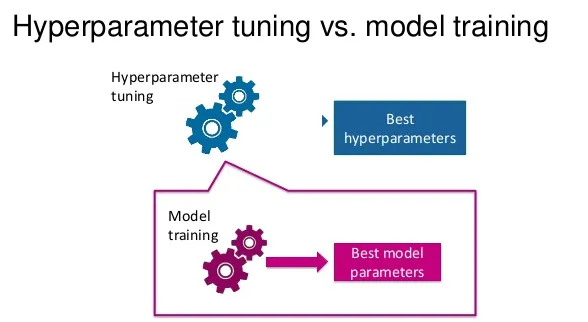Diagram illustrating hyperparameter optimization process

## What is Hyperparameter Optimization?

With its vast potential and intricate mechanisms, machine learning often hinges on fine details. One such detail pivotal to the success of a model is hyperparameter optimization. At its core, this process systematically searches for the best set of hyperparameters to elevate a model's performance.

But what distinguishes hyperparameters from model parameters? Model parameters are the model's aspects learned from the data during training, such as weights in a neural network. Hyperparameters, on the other hand, are set before training begins. They dictate the overarching structure and behavior of a model. They are adjusted settings or dials to optimize the learning process. This includes the learning rate, which determines how quickly a model updates its parameters in response to the training data, or the regularization term, which helps prevent overfitting.4

The challenge of hyperparameter optimization is monumental. Given the vastness of the hyperparameter space, with an almost infinite number of combinations, finding the optimal set is like searching for a needle in a haystack.

Techniques such as grid search, where a predefined set of hyperparameters is exhaustively tried, or random search, where hyperparameters are randomly sampled, are often employed. More advanced methods like Bayesian optimization, which builds a probabilistic model of the function mapping from hyperparameter values to the objective value, are also gaining traction.5

Build Better Models, Faster with Encord's Leading Annotation Tool

### Why Fine-tuning is Essential

The configuration and hyperparameter tuning can profoundly influence a model's performance. A slight tweak can be the difference between a mediocre outcome and stellar results. For instance, the Adam optimizer, a popular **optimization method** in deep learning, has specific hyperparameters that, when fine-tuned, can lead to faster and more stable convergence during training. 6

In real-world applications, hyperparameter search and fine-tuning become even more evident. Consider a scenario where a pre-trained neural network, initially designed for generic image recognition, is repurposed for a specialized task like medical image analysis. Its accuracy and reliability can be significantly enhanced by searching for optimal hyperparameters and fine-tuning them for this dataset. This could mean distinguishing between accurately detecting a medical anomaly and missing it altogether.

Furthermore, as machine learning evolves, our datasets and challenges become more complex. In such a landscape, the ability to fine-tune models and optimize hyperparameters using various optimization methods is not just beneficial; it's essential. It ensures that our models are accurate, efficient, adaptable, and ready to tackle the challenges of tomorrow.

## Techniques for Hyperparameter Optimization

Hyperparameter optimization focuses on finding the optimal set of hyperparameters for a given model. Unlike model parameters, these hyperparameters are not learned during training but are set before the training begins. Their correct setting can significantly influence the model's performance.

### Grid Search

Grid Search involves exhaustively trying out every possible combination of hyperparameters in a predefined search space. For instance, if you're fine-tuning a model and considering two hyperparameters, learning rate and batch size, a grid search would test all combinations of the values you specify for these hyperparameters.

Let's consider classifying images of handwritten digits (a classic problem known as the MNIST classification). Here, the images are 28x28 pixels, and the goal is to classify them into one of the ten classes (0 through 9).

For an SVM applied to this problem, two critical hyperparameters are:

• The type and parameters of the kernel: For instance, if using the Radial Basis Function (RBF) kernel, we need to determine the gamma value.
• The regularization parameter (C) determines the trade-off between maximizing the margin and minimizing classification error.

Using grid search, we can systematically explore combinations of:

• Different kernels: linear, polynomial, RBF, etc.
• Various values of gamma (for RBF): e.g., [0.1, 1, 10, 100]
• Different values of C: e.g., [0.1, 1, 10, 100]

By training the SVM with each combination and validating its performance on a separate dataset, grid search allows us to pinpoint the combination that yields the best classification accuracy.

• Comprehensive: Since it tests all possible combinations, there's a high chance of finding the optimal set.
• Simple to implement: It doesn't require complex algorithms or techniques.

• Computationally expensive: As the number of hyperparameters or their potential values increases, the number of combinations to test grows exponentially.
• Time-consuming: Due to its exhaustive nature, it can be slow, especially with large datasets or complex models.

### Random Search

Random Search, as the name suggests, involves randomly selecting and evaluating combinations of hyperparameters. Unlike Grid Search, which exhaustively tries every possible combination, Random Search samples a predefined number of combinations from a specified distribution for each hyperparameter. 11

Consider a scenario where a financial institution develops a machine learning model to predict loan defaults. The dataset is vast, with numerous features ranging from a person's credit history to current financial status.

The model in question, a deep neural network, has several hyperparameters like learning rate, batch size, and the number of layers. Given the high dimensionality of the hyperparameter space, using Grid Search might be computationally expensive and time-consuming. By randomly sampling hyperparameter combinations, the institution can efficiently narrow down the best settings with the highest prediction accuracy, saving time and computational resources.13

• Efficiency: Random Search can be more efficient than Grid Search, especially when the number of hyperparameters is large. It doesn't need to try every combination, which can save time.12
• Flexibility: It allows for a more flexible specification of hyperparameters, as they can be drawn from any distribution, not just a grid.
• Surprising Results: Sometimes, Random Search can stumble upon hyperparameter combinations that might be overlooked in a more structured search approach.

• No Guarantee: There's no guarantee that Random Search will find the optimal combination of hyperparameters, especially if the number of iterations is too low.
• Dependence on Iterations: The effectiveness of Random Search is highly dependent on the number of iterations. Too few iterations might miss the optimal settings, while too many can be computationally expensive.

### Bayesian Optimization

Bayesian Optimization is a probabilistic model-based optimization technique particularly suited for optimizing expensive-to-evaluate and noisy functions. Unlike random or grid search, Bayesian Optimization builds a probabilistic model of the objective function. It uses it to select the most promising hyperparameters to evaluate the true objective function.

Bayesian Optimization shines in scenarios where the objective function is expensive to evaluate. For instance, training a model with a particular set of hyperparameters in deep learning can be time-consuming. Using grid search or random search in such scenarios can be computationally prohibitive. By building a model of the objective function, Bayesian Optimization can more intelligently sample the hyperparameter space to find the optimal set in fewer evaluations.

Bayesian Optimization is more directed than grid search, which exhaustively tries every combination of hyperparameters, or random search, which samples them randomly. It uses past evaluation results to choose the next set of hyperparameters to evaluate. This makes it particularly useful when evaluating the objective function (like training a deep learning model) is time-consuming or expensive.

However, it's worth noting that if the probabilistic model's assumptions do not align well with the true objective function, Bayesian Optimization might not perform as well. A more naive approach like random search might outperform it in such cases.1

• Efficiency: Bayesian Optimization typically requires fewer function evaluations than random or grid search, making it especially useful for optimizing expensive functions.
• Incorporation of Prior Belief: It can incorporate prior beliefs about the function and then sequentially refine this model as more samples are collected.
• Handling of Noisy Objective Functions: It can handle noisy objective functions, meaning that there's some random noise added to the function's output each time it's evaluated.

• Model Assumptions: The performance of Bayesian Optimization can be sensitive to the assumptions made by the probabilistic model.
• Computationally Intensive: As the number of observations grows, the computational complexity of updating the probabilistic model and selecting the next sample point can become prohibitive.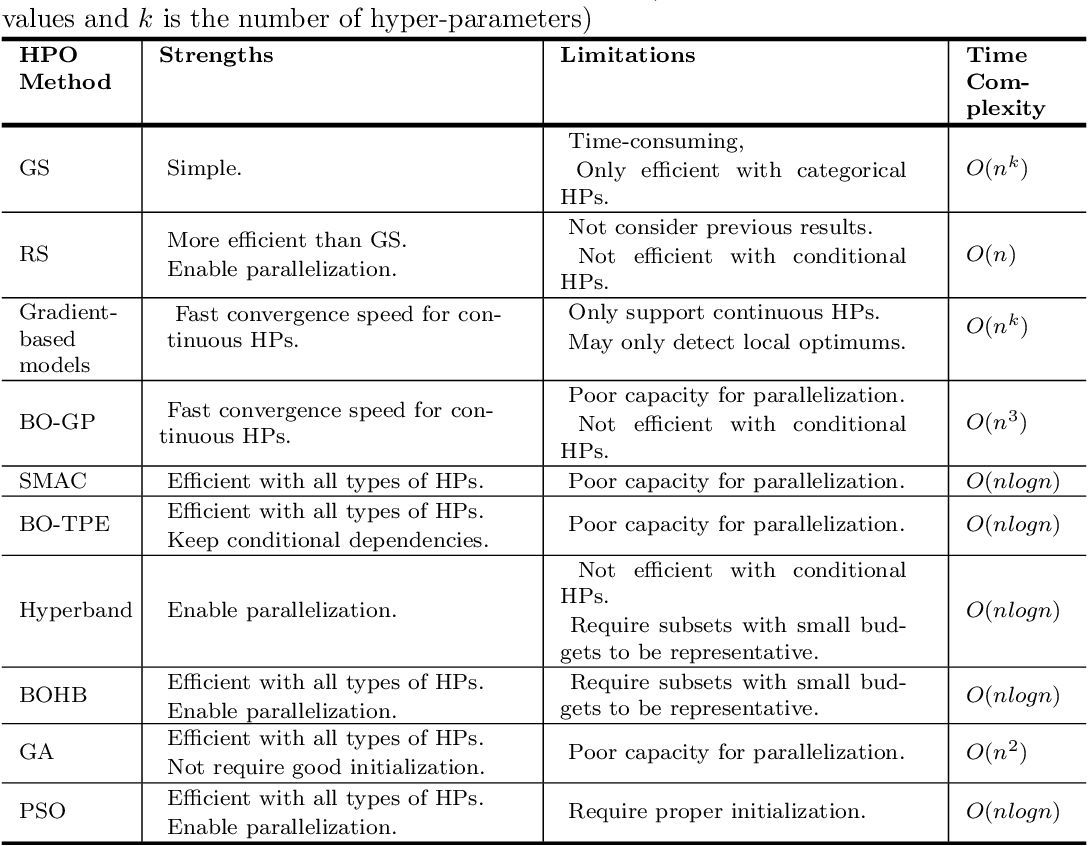A comparison chart of different optimization techniques

## The Role of Adam Optimizer Hyperparameters

The Adam optimizer has emerged as a popular choice for training deep learning models in the vast landscape of optimization algorithms. But what makes it so special? And how do its hyperparameters influence the fine-tuning process?

### Introduction to the Adam Optimizer

The Adam optimizer, short for Adaptive Moment Estimation, is an optimization algorithm for training neural networks. It combines two other popular optimization techniques: AdaGrad and RMSProp. The beauty of Adam is that it maintains separate learning rates for each parameter and adjusts them during training. This adaptability makes it particularly effective for problems with sparse gradients, such as natural language processing tasks.5

### Significance of Adam in Model Training

Adam has gained popularity due to its efficiency and relatively low memory requirements. Unlike traditional gradient descent, which maintains a single learning rate for all weight updates, Adam computes adaptive learning rates for each parameter. This means it can fine-tune models faster and often achieve better performance on test datasets. Moreover, Adam is less sensitive to hyperparameter settings, making it a more forgiving choice for those new to model training.. 7

### Impact of Adam's Hyperparameters on Model Training

The Adam optimizer has three primary hyperparameters: the learning rate, beta1, and beta2. Let's break down their roles:

• Learning Rate (α): This hyperparameter determines the step size at each iteration while moving towards a minimum in the loss function. A smaller learning rate might converge slowly, while a larger one might overshoot the minimum.
• Beta1: This hyperparameter controls the exponential decay rate for the first-moment estimate. It's essentially a moving average of the gradients. A common value for beta1 is 0.9, which means the algorithm retains 90% of the previous gradient's value.8
• Beta2 controls the exponential decay rate for the second moment estimate, an uncentered moving average of the squared gradient. A typical value is 0.999. 8

Fine-tuning these hyperparameters can significantly impact model training. For instance, adjusting the learning rate can speed up convergence or prevent the model from converging. Similarly, tweaking beta1 and beta2 values can influence how aggressively the model updates its weights in response to the gradients.

### Practical Tips for Fine-tuning with Adam

• Start with a Smaller Learning Rate: While Adam adjusts the learning rate for each parameter, starting with a smaller global learning rate (e.g., 0.0001) can lead to more stable convergence, especially in the early stages of training.
• Adjust Beta Values: The default values of beta1 = 0.9 and beta2 = 0.999 work well for many tasks. However, slightly adjusting specific datasets or model architectures can lead to faster convergence or better generalization.
• Monitor Validation Loss: Always monitor your validation loss. If it starts increasing while the training loss continues to decrease, it might be a sign of overfitting. Consider using early stopping or adjusting your learning rate.
• Warm-up Learning Rate: Gradually increasing the learning rate at the beginning of training can help stabilize the optimizer. This "warm-up" phase can prevent large weight updates that can destabilize the model early on.
• Use Weight Decay: Regularization techniques like weight decay can help prevent overfitting, especially when training larger models or when the dataset is small.
• Epsilon Value: While the default value of ε is usually sufficient, increasing it slightly can help with numerical stability in some cases.

### Best Practices

• Learning Rate Scheduling: Decreasing the learning rate as training can help achieve better convergence. Techniques like step decay or exponential decay can be beneficial.
• Batch Normalization: Using batch normalization layers in your neural network can make the model less sensitive to the initialization of weights, aiding in faster and more stable training.
• Regular Checkpoints: Always save model checkpoints regularly. This helps in unexpected interruptions and allows you to revert to a previous state if overfitting occurs.

Adam optimizer is powerful and adaptive; understanding its intricacies and fine-tuning its hyperparameters can improve model performance. Following these practical tips and best practices ensures that your model trains efficiently and generalizes well to unseen data.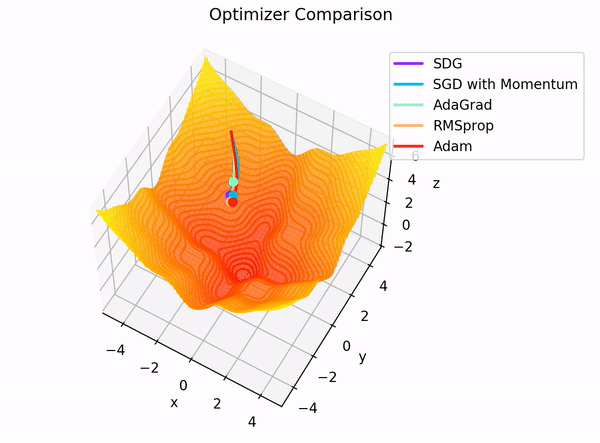Visualization of Adam Optimizer in action

## Challenges in Hyperparameter Optimization

Let's delve into common pitfalls practitioners face while choosing the best hyperparameters and explore strategies to overcome them.

### The Curse of Dimensionality

When dealing with many hyperparameters, the search space grows exponentially. This phenomenon, known as the curse of dimensionality, can make the optimization process computationally expensive and time-consuming. 9

Strategy: One way to tackle this is by using dimensionality reduction techniques or prioritizing the most impactful hyperparameters.

### Local Minima and Plateaus

Optimization algorithms can sometimes get stuck in local minima or plateaus, where further adjustments to hyperparameters don't significantly improve performance. 10

Strategy: Techniques like random restarts, where the optimization process is started from different initial points, or using more advanced optimization algorithms like Bayesian optimization, can help navigate these challenges.

### Overfitting

Strategy: Regularization techniques, cross-validation, and maintaining a separate validation set can help prevent overfitting during hyperparameter optimization.

{{grey_callout_start}} For a deeper dive into data splitting techniques crucial for segregating the training set and test set, and playing a pivotal role in model training and validation, check out our detailed article on Train-Validation-Test Split. {{grey_callout_end}}

### Computational Constraints

Hyperparameter optimization, especially grid search, can be computationally intensive. This becomes a challenge when resources are limited.

Strategy: Opt for more efficient search methods like random or gradient-based optimization, which can provide good results with less computational effort.

### Lack of Clarity on Which Hyperparameters to Tune

Strategy: Start with the most commonly tuned hyperparameters. For instance, when fine-tuning models using the Adam optimizer, focus on learning and decay rates. 9

Hyperparameter optimization is essential for achieving the best model performance, and awareness of its challenges is crucial. By understanding these challenges and employing the strategies mentioned, practitioners can navigate the optimization process more effectively and efficiently.

### Overfitting and Regularization

#### The Balance Between Model Complexity and Generalization

A model's complexity is directly related to its number of parameters. While a more complex model can capture intricate patterns in the training data, it's also more susceptible to overfitting.4 Conversely, a too-simple model might not capture the necessary patterns, leading to underfitting. The challenge lies in finding the sweet spot where the model is complex enough to learn from the training data but not so much that it loses its generalization ability.

#### Role of Hyperparameters in Preventing Overfitting

Hyperparameters can significantly influence a model's complexity. For instance, the number of layers and nodes in neural networks can determine how intricate patterns the model can capture.9 However, we can fine-tune this balance with the Adam optimizer and its hyperparameters.

The learning rate, one of the primary hyperparameters of the Adam optimizer, determines the step size at each iteration while moving towards a minimum of the loss function. A lower learning rate might make the model converge slowly, but it can also help avoid overshooting and overfitting. On the other hand, a larger learning rate might speed up the convergence. Still, it can cause the model to miss the optimal solution. 9

Regularization techniques, like L1 and L2 regularization, add a penalty to the loss function. By adjusting the regularization hyperparameter, one can control the trade-off between fitting the training data closely and keeping the model weights small to prevent overfitting.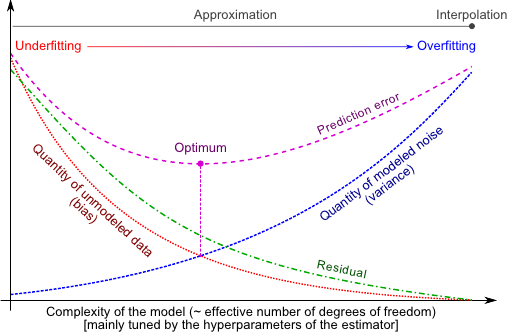Graph illustrating overfitting and the role of hyperparameters

## Hyperparameter Optimization: Key Takeaways

In the intricate landscape of machine learning, hyperparameter tuning and hyperparameter search are essential processes, ensuring that models achieve optimal performance through meticulous fine-tuning. The balance between model complexity and its generalization capability is paramount. The role of hyperparameters, especially within the framework of the Adam optimizer, is pivotal in maintaining this equilibrium and finding the optimal hyperparameters.

As machine learning continues to evolve, practitioners must remain aware of evolving methodologies and optimization methods. The hyperparameter optimization process is not a mere task but an ongoing commitment to refining models for superior outcomes.

It is, therefore, incumbent upon professionals in this domain to engage in rigorous experimentation and continual learning, ensuring that the models they develop are efficient, robust, and adaptable to the ever-evolving challenges presented by real-world data.

Scale your annotation workflows and power your model performance with data-driven insights#### The Complete Data Engine for AI Model Development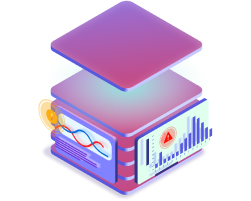###### Subscribe